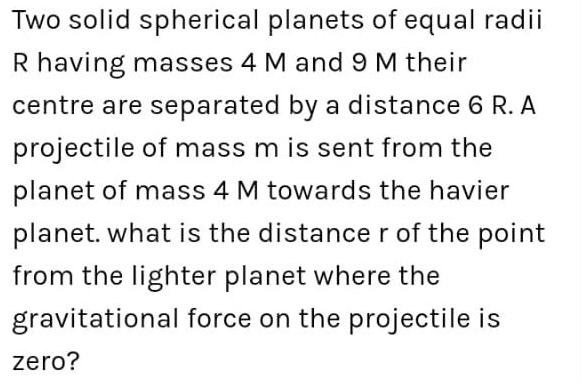Question:

# Two solid spherical planets of equal radii R having masses 4

Last updated: 7/31/2022Two solid spherical planets of equal radii R having masses 4 M and 9 M their centre are separated by a distance 6 R. A projectile of mass m is sent from the planet of mass 4 M towards the havier planet. what is the distance r of the point from the lighter planet where the gravitational force on the projectile is zero?